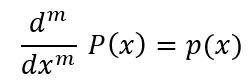GeeksforGeeks App
Open AppBrowser
Continue

# numpy.polyint() in Python

`numpy.polyint(p, m) `: Evaluates the anti – derivative of a polynomial with the specified order.

m antiderivative ‘P’ of polynomial ‘p’ satisfiesParameters :
p : [array_like or poly1D] polynomial coefficients are given in decreasing order of powers. If the second parameter (root) is set to True then array values are the roots of the polynomial equation. For example, poly1d(3, 2, 6) = 3x2 + 2x + 6
m : [int, optional] Order of anti-derivative. Default is 1.

Return: Anti-Derivative of the polynomial.

Code #1:

 `# Python code explaining  ``# numpy.polyint() ``     ` `# importing libraries ``import` `numpy as np ``   ` `# Constructing polynomial  ``p1 ``=` `np.poly1d([``1``, ``2``])  ``p2 ``=` `np.poly1d([``4``, ``9``, ``5``, ``4``])  ``     ` `print` `(``"P1 : "``, p1)  ``print` `(``"\n p2 : \n"``, p2) `` ` `# Solve for x = 2  ``print` `(``"\n\np1 at x = 2 : "``, p1(``2``))  ``print` `(``"p2 at x = 2 : "``, p2(``2``))  `` ` `a ``=` `np.polyint(p1, ``1``) ``b ``=` `np.polyint(p2, ``1``) ``print` `(``"\n\nUsing polyint"``) ``print` `(``"p1 anti-derivative of order = 1 : \n"``, a) ``print` `(``"p2 anti-derivative of order = 1 : \n"``, b) `` ` `a ``=` `np.polyint(p1, ``2``) ``b ``=` `np.polyint(p2, ``2``) ``print` `(``"\n\nUsing polyint"``) ``print` `(``"p1 anti-derivative of order = 2 : \n"``, a) ``print` `(``"p2 anti-derivative of order = 2 : \n"``, b) `

Output :

```P1 :
1 x + 2

p2 :
3     2
4 x + 9 x + 5 x + 4

p1 at x = 2 :  4
p2 at x = 2 :  82

Using polyint
p1 anti-derivative of order = 1 :
2
0.5 x + 2 x
p2 anti-derivative of order = 1 :
4     3       2
1 x + 3 x + 2.5 x + 4 x
```

Code #2:

 `# Python code explaining  ``# numpy.polyint() ``      ` `# importing libraries ``import` `numpy as np ``    ` `# Constructing polynomial  ``p1 ``=` `np.poly1d([``1``, ``2``])  ``p2 ``=` `np.poly1d([``4``, ``9``, ``5``, ``4``])  ``    ` `a ``=` `np.polyint(p1, ``2``) ``b ``=` `np.polyint(p2, ``2``) `` ` `print` `(``"\n\nUsing polyint"``) ``print` `(``"p1 anti-derivative of order = 2 : \n"``, a) ``print` `(``"p2 anti-derivative of order = 2 : \n"``, b) `

Output :

```Using polyint
p1 anti-derivative of order = 2 :
3     2
0.1667 x + 1 x
p2 anti-derivative of order = 2 :
5        4          3     2
0.2 x + 0.75 x + 0.8333 x + 2 x```

My Personal Notes arrow_drop_up